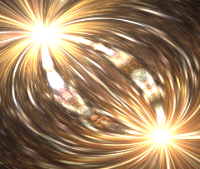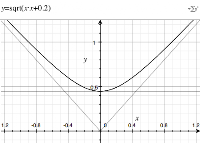## Friday, June 17, 2011

### Deform (textured interference) effect in WebGL'deform' interference effect
This effect is one of my all time favourites. It is somewhat similar to the XOR bitplasma effect with which I started the tutorial series based on iq's shader toy. This effect is an 'interference' of two points based on the subtraction of the radii and angles.

Jumping straight to the solution; the code we are trying to decipher is:
``` float a1 = atan(p.y-offset1.y,p.x-offset1.x); float r1 = sqrt(dot(p-offset1,p-offset1)); float a2 = atan(p.y-offset2.y,p.x-offset2.x); float r2 = sqrt(dot(p-offset2,p-offset2)); vec2 uv; uv.x =(r1-r2); uv.y =(a1-a2);```
` `r1

So why does it look the way it looks?r2

Lets start with the radius part. If we just look at one radius (r1) we would expect to see a steadily increasing value radiating out from our offset point (in all my examples this will be at 0.3)

And this is exactly what we see when we plot this. If we plot the inverse (negative r2, offset to -0.3) we should see the inverse, that is, a steadily decreasing value radiating from the offset point.r1-r2

So if we combine these (r1-r2) we get a nice smooth surface transition between these two patterns. Where does this blending come from?

Lets take it back a step and look at our problem in just one dimension. Our definition of the radius is sqrt(x*x+y*y), in 1D we can plot x*x and sqrt(x*x):

Coming back to the generation of the surface, we can plot two interfering 1D functions, that is sqrt((x-0.3)*(x-0.3)) and sqrt((x+0.3)*(x+0.3)), and the difference (i.e. the first subtract the second). This gives us a ramped step between the functions as each reaches its turning point.

We can see the same feature in the surface, if we view it from an appropriate angle.r1-r2, alternative viewWe still haven't discovered the cause of the smooth blending that gives such a nice visual effect. When we add a constant (i.e. offset) to the function sqrt(x*x+k), notice how we now have the turning point at sqrt(k) and have a parabola instead. This is effectively evaluating sqrt(x*x+y*y) for some constant y=sqrt(k).

So, if we now consider the two extremes, again plotting in 1D but considering the 2D case for both our x-offsets of =-0.3 and 0.3 we get a set of blended curves (dark blue/red is y=-0.3, light blue/purple is y = 0.3).

And that is where our full surface comes from.Left: atan(x) Right: atan(1/y)
Now we want to understand the blend in the angles. The generating function is nothing more complex than atan(x/y). Below is a graph of atan(x), followed by atan(1/y). Note: There are different viewing angles for each function so it is easier to see the curvature.

If we combine these two equations into one (i.e. atan(x/y)) we get:atan(x/y)

Now, we just need to understand the interference pattern that is generated from two of these functions interacting. I'm going to take a shortcut here and just let an animated gif tell the story. This animation shows a discretized version of the angles (first in steps of ~60 degrees, then ~30, etc.) interacting for the equation a1-a2 from the code snippet.a1-a2 discretized and animated (open as a separate image)

From the gif you can see how the curves evolve. To understand these better we can take a look at how this curve pattern comes about for a constant difference angle. If we modify the end of our original shader code to:
``` uv.y = (a2-a1); float k = 0.0; if ((uv.y > 0.3) && (uv.y<0.31)) k = 1.0; gl_FragColor = vec4(col.r+k,col.g+k,col.b+k,1.0); ```

The resulting image is still the original effect, but one of the curves is highlighted (in white). This represents a curve where (a2-a1) ~= 0.3

We can understand this curve by generating a set of triangles in which two vertices are fixed, and the difference between two angles is held constant. By altering one angle the final vertex will generate the sweeping curve in the original image (See Law of Sines). In the example below, we vary a1 and hold a2 as (a1 - 30 degrees).

This generates our final explanatory image of the sweeping triangles:

Finally! The full effect has been deciphered, click the button below to see it in action. (You will need a WebGL enabled browser)

Your browser doesn't appear to support the HTML5 `<canvas>` element.

Giles said...

On FF5 I get

Error: uncaught exception: [Exception... "Failure arg 5 [nsIDOMWebGLRenderingContext.texImage2D]" nsresult: "0x80004005 (NS_ERROR_FAILURE)" location: "JS frame :: http://adrianboeing.blogspot.com/2011/06/deform-textured-interference-effect-in.html :: handleLoadedTexture :: line 682" data: no]

Seems to work on Chrome though.

Benoit Jacob said...

This script is trying to use a cross-domain image as the source for a WebGL texture, that's why it doesn't work anymore in Firefox >= 5. The JS error console has a warning explaining that:

Warning: WebGL: It is forbidden to load a WebGL texture from a cross-domain element. See https://developer.mozilla.org/en/WebGL/Cross-Domain_Textures